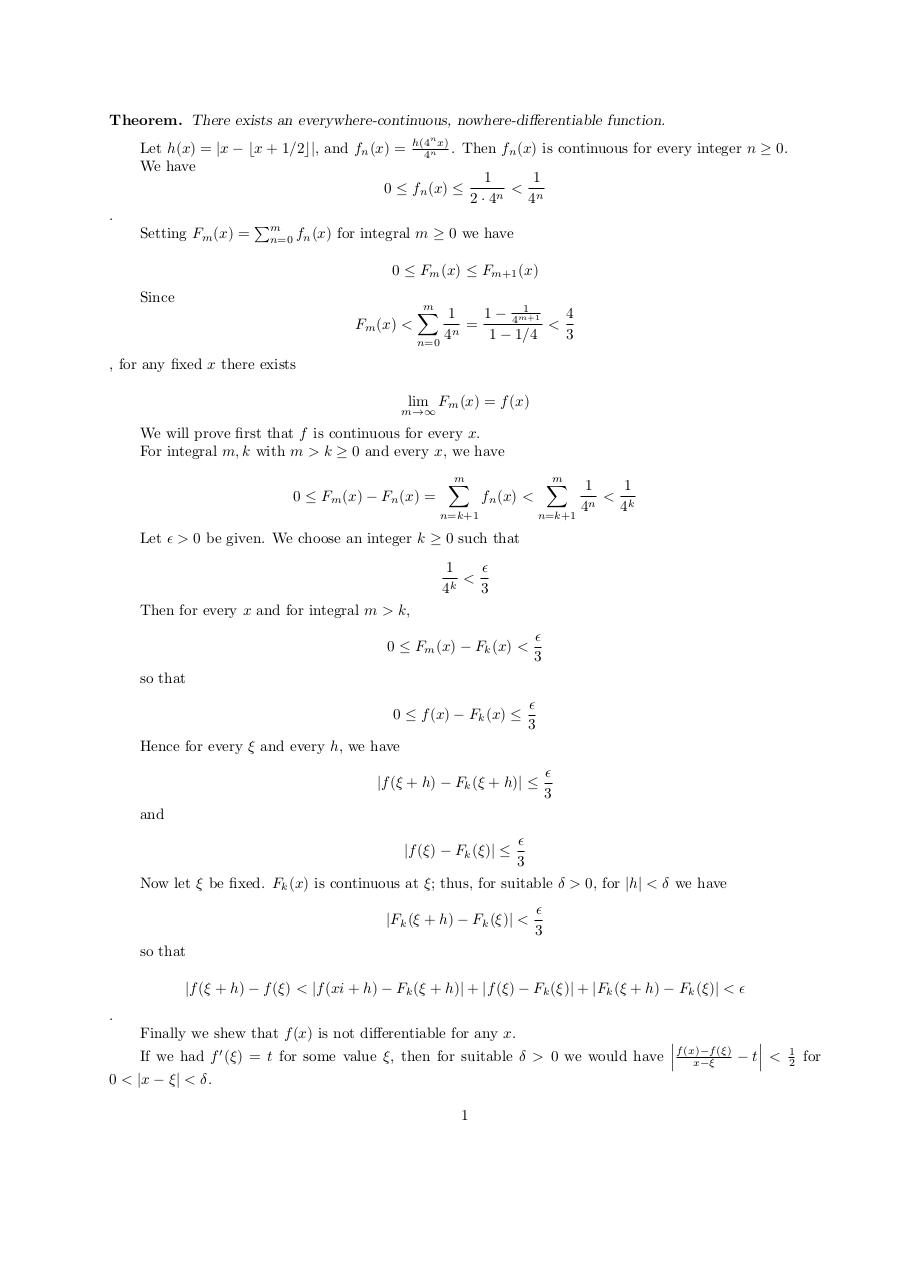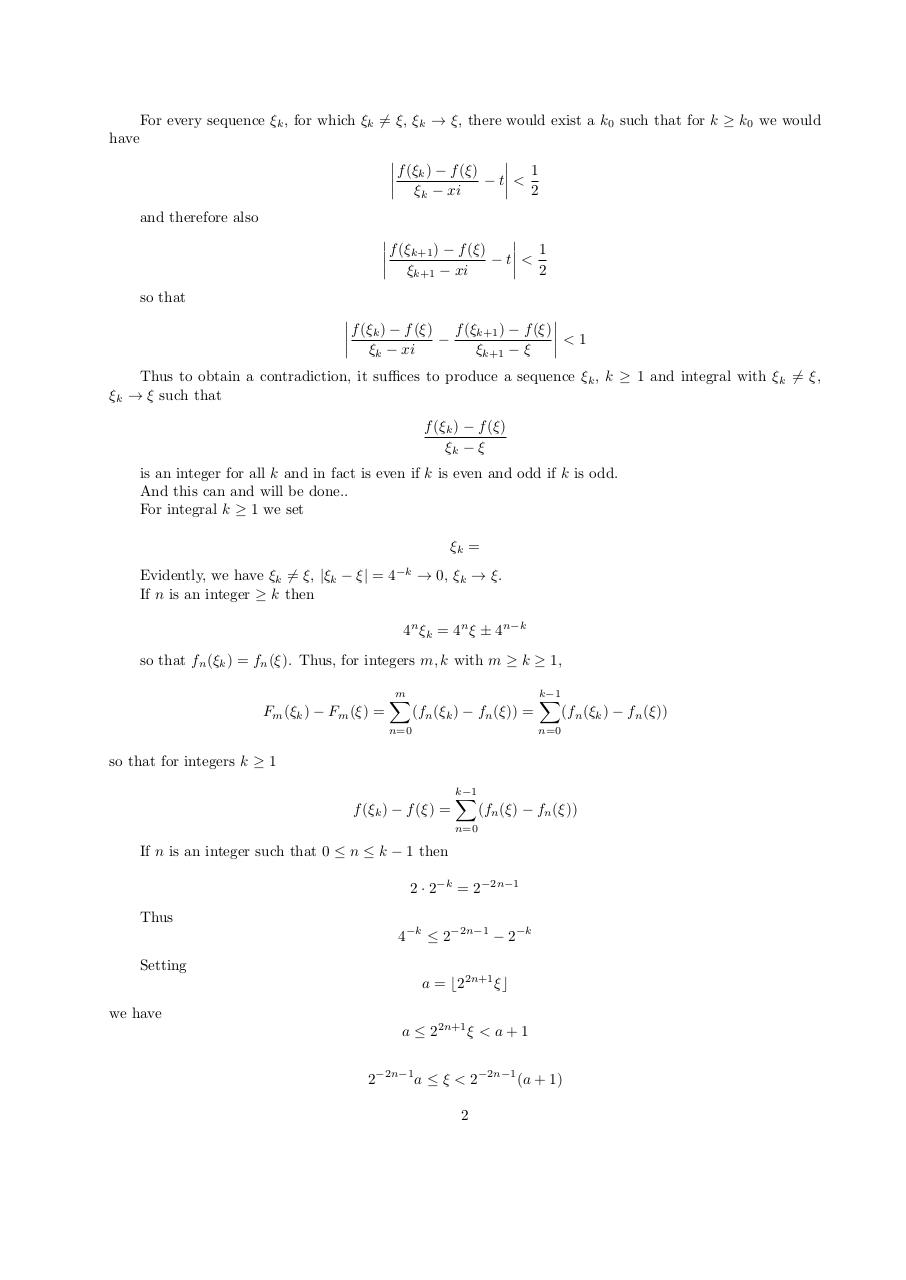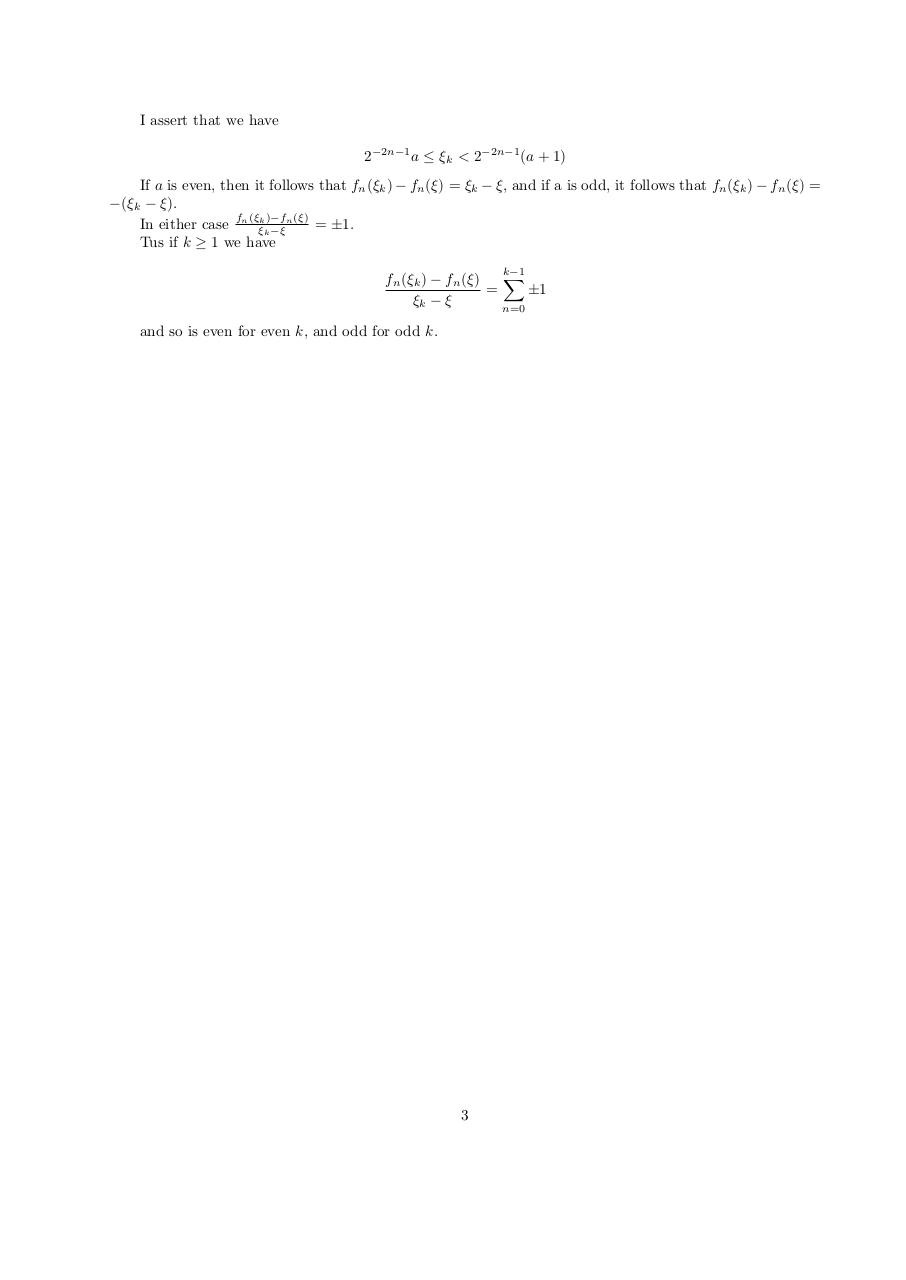# nodiff .pdf

### File information

Original filename: nodiff.pdf

This PDF 1.5 document has been generated by TeX / pdfTeX-1.40.17, and has been sent on pdf-archive.com on 29/05/2017 at 18:51, from IP address 172.8.x.x. The current document download page has been viewed 214 times.
File size: 113 KB (3 pages).
Privacy: public file

## Download original PDF file

nodiff.pdf (PDF, 113 KB)

### Document preview

Theorem. There exists an everywhere-continuous, nowhere-differentiable function.
n

Let h(x) = |x − bx + 1/2c|, and fn (x) = h(44n x) . Then fn (x) is continuous for every integer n ≥ 0.
We have
1
1
&lt; n
0 ≤ fn (x) ≤
n
2·4
4
.
Setting Fm (x) =

Pm

n=0

fn (x) for integral m ≥ 0 we have
0 ≤ Fm (x) ≤ Fm+1 (x)

Since

m
1
X
1 − 4m+1
1
4
Fm (x) &lt;
=
&lt;
n
4
1

1/4
3
n=0

, for any fixed x there exists
lim Fm (x) = f (x)

m→∞

We will prove first that f is continuous for every x.
For integral m, k with m &gt; k ≥ 0 and every x, we have
0 ≤ Fm (x) − Fn (x) =

m
X

m
X

fn (x) &lt;

n=k+1

n=k+1

1
1
&lt; k
n
4
4

Let &gt; 0 be given. We choose an integer k ≥ 0 such that

1
&lt;
4k
3
Then for every x and for integral m &gt; k,
0 ≤ Fm (x) − Fk (x) &lt;

3

so that
0 ≤ f (x) − Fk (x) ≤

3

Hence for every ξ and every h, we have
|f (ξ + h) − Fk (ξ + h)| ≤

3

and

3
Now let ξ be fixed. Fk (x) is continuous at ξ; thus, for suitable δ &gt; 0, for |h| &lt; δ we have
|f (ξ) − Fk (ξ)| ≤

|Fk (ξ + h) − Fk (ξ)| &lt;

3

so that
|f (ξ + h) − f (ξ) &lt; |f (xi + h) − Fk (ξ + h)| + |f (ξ) − Fk (ξ)| + |Fk (ξ + h) − Fk (ξ)| &lt;
.
Finally we shew that f (x) is not differentiable for any x.

(ξ)
If we had f 0 (ξ) = t for some value ξ, then for suitable δ &gt; 0 we would have f (x)−f

t
&lt;
x−ξ
0 &lt; |x − ξ| &lt; δ.
1

1
2

for

For every sequence ξk , for which ξk 6= ξ, ξk → ξ, there would exist a k0 such that for k ≥ k0 we would
have

f (ξk ) − f (ξ)
1

− t &lt;
ξk − xi
2
and therefore also

f (ξk+1 ) − f (ξ)
1

− t &lt;
ξk+1 − xi
2
so that

f (ξk ) − f (ξ) f (ξk+1 ) − f (ξ)

&lt;1

ξk − xi

ξk+1 − ξ
Thus to obtain a contradiction, it suffices to produce a sequence ξk , k ≥ 1 and integral with ξk 6= ξ,
ξk → ξ such that
f (ξk ) − f (ξ)
ξk − ξ
is an integer for all k and in fact is even if k is even and odd if k is odd.
And this can and will be done..
For integral k ≥ 1 we set
ξk =
Evidently, we have ξk 6= ξ, |ξk − ξ| = 4−k → 0, ξk → ξ.
If n is an integer ≥ k then
4n ξk = 4n ξ ± 4n−k
so that fn (ξk ) = fn (ξ). Thus, for integers m, k with m ≥ k ≥ 1,
Fm (ξk ) − Fm (ξ) =

m
X

(fn (ξk ) − fn (ξ)) =

n=0

k−1
X

(fn (ξk ) − fn (ξ))

n=0

so that for integers k ≥ 1
f (ξk ) − f (ξ) =

k−1
X

(fn (ξ) − fn (ξ))

n=0

If n is an integer such that 0 ≤ n ≤ k − 1 then
2 · 2−k = 2−2n−1
Thus
4−k ≤ 2−2n−1 − 2−k
Setting
a = b22n+1 ξc
we have
a ≤ 22n+1 ξ &lt; a + 1
2−2n−1 a ≤ ξ &lt; 2−2n−1 (a + 1)
2

I assert that we have
2−2n−1 a ≤ ξk &lt; 2−2n−1 (a + 1)
If a is even, then it follows that fn (ξk ) − fn (ξ) = ξk − ξ, and if a is odd, it follows that fn (ξk ) − fn (ξ) =
−(ξk − ξ).
n (ξ)
= ±1.
In either case fn (ξξkk)−f
−ξ
Tus if k ≥ 1 we have
k−1
fn (ξk ) − fn (ξ) X
=
±1
ξk − ξ
n=0

and so is even for even k, and odd for odd k.

3### Link to this page

#### Permanent link

Use the permanent link to the download page to share your document on Facebook, Twitter, LinkedIn, or directly with a contact by e-Mail, Messenger, Whatsapp, Line..

#### Short link

Use the short link to share your document on Twitter or by text message (SMS)

#### HTML Code

Copy the following HTML code to share your document on a Website or Blog

#### QR Code### Related keywords